# The Complex Exponential

in STEMGeeks2 months ago

Hi there. In this post, I talk about the complex exponential function. This topic deals with complex numbers, an extension of the real numbers.

I have a previous post about graphing complex numbers.

As usual, math rendered in LaTeX with QuickLatex.comPixabay Image Source

## Review of Complex Numbers

In the real number system, taking the square root of a negative number gives an error. In the complex numbers space, you can take the square root of a negative number. The square root of negative one is called `i`.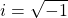There is makes the square of `i` equal to negative one.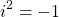A complex number `z` has a real part `a` and an imaginary part `b` of the form: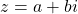For even more notation, `a` can be rewritten as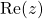for the real part of the complex number `z`. The complex part of the number `z` can be written as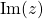for `b`.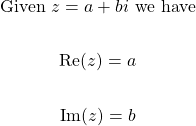Pixabay Image Source

## Polar Form Of Representing Complex Numbers

We can relate complex numbers with trigonometry. I do not want to go into great details on derivations here.

A complex number's modulus |z| or `r` does come from the Pythagorean Theorem.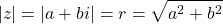The real part of the complex number can also be equal to the radius/modulus multiplied by an angle.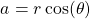The complex part of the complex number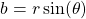These two new formulas for `a` and `b` can be used for a new form for the complex number `z`.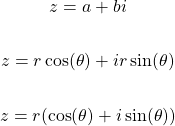The radius is common factored out to obtain the last line. Instead of the last line, some like to adopt the shorthand notation of `cis` for `cosine + i sine of an angle`.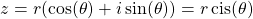Pixabay Image Source

## Complex Exponential

Trigonometric functions can be related to the complex exponential function. Here is Leonard Euler's formula given an angle `theta`.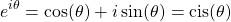The angle `theta` can be obtained with trigonometry. Use the formula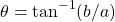. This is the tangent inverse or `arctan` to find the angle of a numeric value.

## Euler's Formula

The famous Euler's famous formula comes from the complex exponential when `x = π` (pi).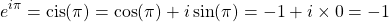Euler's identity can be obtained by moving the negative one over from the right to the left side. The right side is zero.Pixabay Image Source

## Examples Of Working With The Complex Exponential

Example One

Evaluate the complex exponential when the angle theta is `pi/2`.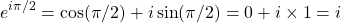Example Two

If the angle in the complex exponential is negative pi we have: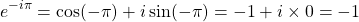Example Three - Product Case

Suppose that two different angles are given such as `pi` and `3pi/2`. The product of the two complex exponentials would be: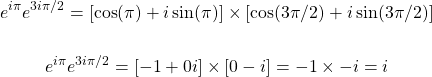This is also equal to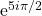if you use exponent laws. This way is faster.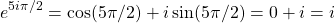If you have two different angles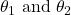, the product of complex exponentials is the complex exponential where the angles are added together.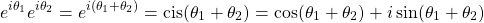The above deals with two angles from two complex exponentials. You can easily extend this to `n` number of angles and use the Sigma sum notation and the product notation.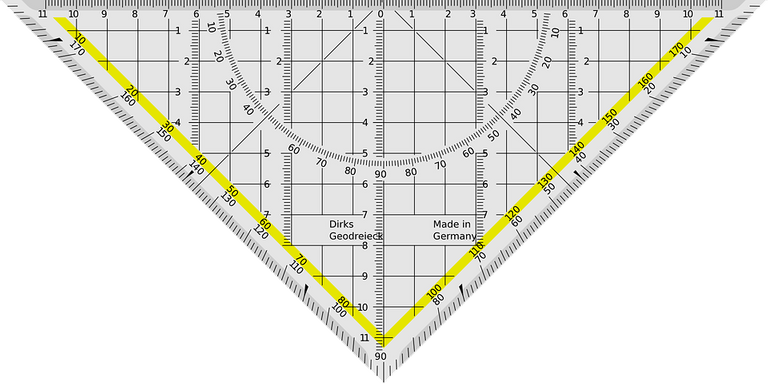Pixabay Image Source